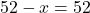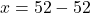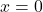## Look at this equation. 3(x + 1) = 3x + Which number can be placed in the box that would create an equation that has an infinite number of so

Question

Look at this equation. 3(x + 1) = 3x + Which number can be placed in the box that would create an equation that has an infinite number of solutions? OA) O OB) 1 C) 2 OD 3​

in progress 0
5 months 2021-08-24T23:21:25+00:00 1 Answers 2 views 0

1.